Home | | Artificial Intelligence | Uninformed Search Strategies

# Uninformed Search Strategies

Uninformed search methods: ŌĆó Breadth-first search ŌĆó Uniform-cost search ŌĆó Depth-first search ŌĆó Depth-limited search ŌĆó Iterative deepening search

UNINFORMED SEARCH STRATEGIES

ŌĆó                      Uninformed strategies use only the information available in the problem definition

ŌĆō  Also known as blind searching

ŌĆō  Uninformed search methods:

ŌĆó                                                                                          Uniform-cost search

ŌĆó                                                                                          Depth-first search

ŌĆó                                                                                          Depth-limited search

ŌĆó                                                                                          Iterative deepening search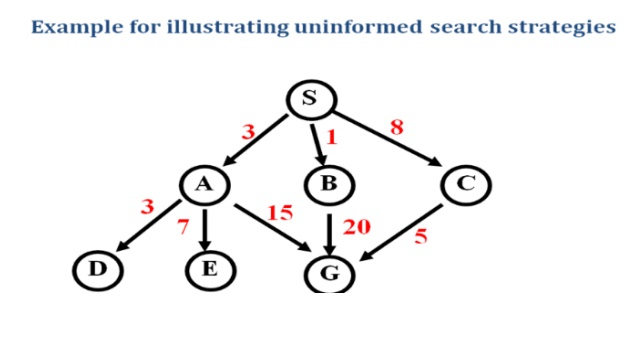FIRSTSEARCH

Definition:

The root node is expanded first, and then all the nodes generated by the node are expanded.

ŌĆó                   Expand the shallowest unexpanded node

ŌĆó                   Place all new successors at the end of a FIFO queue

Implementation: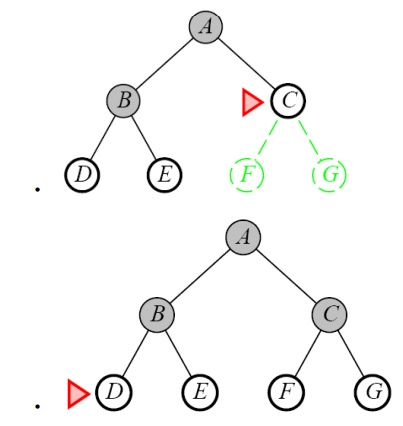ŌĆó                   Complete

ŌĆō  Yes if b (max branching factor) is finite

ŌĆó                   Time

ŌĆō  1 + b + b2 + ŌĆ” + bd + b(bd-1) = O(bd+1)

ŌĆō  exponential in d

ŌĆó                   Space

ŌĆō  O(bd+1)

ŌĆō  Keeps every node in memory

ŌĆō This is the big problem; an agent that generates nodes at 10 MB/sec will produce

860 MB in 24 hours

ŌĆó                   Optimal

ŌĆō  Yes (if cost is 1 per step); not optimal in general

The memory requirements are a bigger problem for breadth-first search than is execution time

ŌĆó                   Exponential-complexity search problems cannot be solved by uniformed methods for any but the smallest instances

Ex: Route finding problem

Given:Task: Find the route from  S to G using BFS.

Step1: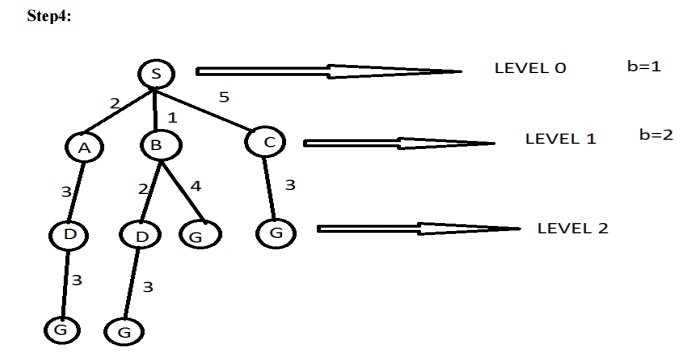Answer : The path in the 2nd depth level that is SBG (or ) SCG.

Time complexityDEPTH-FIRST SEARCH OR BACK TRACKING SEARCHING

Definition:

Expand one node to the depth of the tree. If dead end occurs, backtracking is done to the next immediate previous node for the nodes to be expanded

ŌĆó                      Expand the deepest unexpanded node

ŌĆó                      Unexplored successors are placed on a stack until fully explored

ŌĆó                      Enqueue nodes on nodes in LIFO (last-in, first-out) order. That is, nodes used as a stack data structure to order nodes.

ŌĆó                      It has modest memory requirement.

ŌĆó                      It needs to store only a single path from the root to a leaf node, along with remaining unexpanded sibling nodes for each node on a path

ŌĆó                      Back track uses less memory.

Implementation: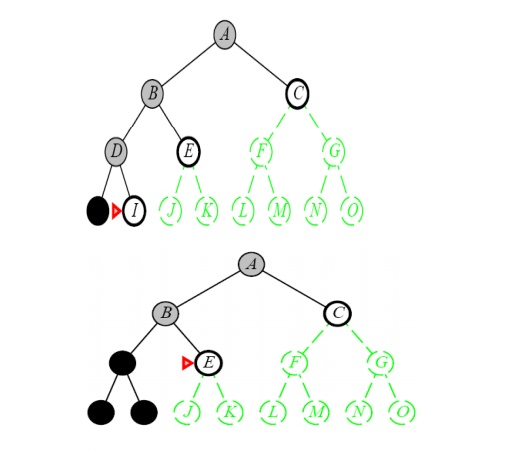Properties of Depth-First Search

ŌĆó                   Complete

ŌĆō  No: fails in infinite-depth spaces, spaces with loops

ŌĆó                                                                 Modify to avoid repeated spaces along path

ŌĆō  Yes: in finite spaces

ŌĆó                   Time

ŌĆō  O(bm)

ŌĆō  Not great if m is much larger than d

ŌĆō  But if the solutions are dense, this may be faster than breadth-first search

ŌĆó                   Space

ŌĆō  O(bm)ŌĆ”linear space

ŌĆó                   Optimal

ŌĆō  No

ŌĆó                   When search hits a dead-end, can only back up one level at a time even if the ŌĆ£problemŌĆØ occurs because of a bad operator choice near the top of the tree. Hence, only does ŌĆ£chronological backtrackingŌĆØ

ŌĆó                   If more than one solution exists or no of levels is high then dfs is best because exploration is done only a small portion of the white space.

ŌĆó                     No guaranteed to find solution.

Example:  Route finding problem

Given problem: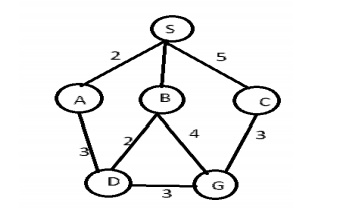Task: Find a route between A to B

Step 1: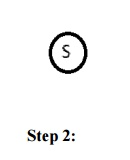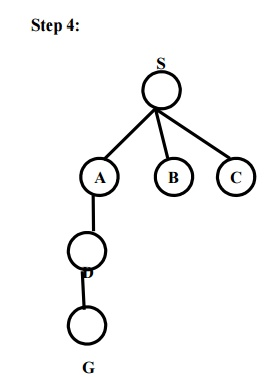DEPTH-LIMITED SEARCH

A cut off (Maximum level of the depth) is introduced in this search technique to overcome the disadvantage of Depth First Sea rch. The cut off value depends on the number o f states.DLS can be implemented as a simple modification to the general tree search algo rithm or the recursive DFS algorithm.DLS im poses a fixed depth limit on a dfs.

A variation of depth-first searc h that uses a depth limit

ŌĆō  Alleviates the problem of unbounded trees

ŌĆō  Search to a predet ermined depth l (ŌĆ£ellŌĆØ)

ŌĆō  Nodes at depth l h ave no successors

ŌĆó                      Same as depth-first search if l = Ōł×

ŌĆó                      Can terminate for failure and cutoff

ŌĆó                      Two kinds of failure

Standard failure: indicates no solution

Cut off: indicates no solution within the depth limitProperties of Depth-Limited Search

ŌĆó                      Complete

ŌĆō  Yes if l < dŌĆó                      Time

ŌĆō  N(IDS)=(d)b+(d-1)b┬▓+ŌĆ”ŌĆ”ŌĆ”ŌĆ”ŌĆ”ŌĆ”ŌĆ”ŌĆ”..+(1)

ŌĆó                   Space

ŌĆō  O(bl)

ŌĆó                   Optimal

ŌĆō  No if l > d

ŌĆó                   Cut off level is introduced in DFS Technique.

ŌĆó                   No guarantee to find the optimal solution.

E.g.: Route finding problem

Given:The number of states in the given map is five. So it is possible to get the goal state at the maximum depth of four. Therefore the cut off value is four.

Task: find a path from A to E.ITERATIVE DEEPENING SEARCH (OR) DEPTH-FIRST ITERATIVE DEEPENING (DFID):

Definition:

ŌĆó                      Iterative deepening depth-first search It is a strategy that steps the issue of choosing the best path depth limit by trying all possible depth limit

Uses depth-first search

Finds the best depth limit

Gradually increases the depth limit; 0, 1, 2, ŌĆ” until a goal is found

Iterative Lengthening Search:

The idea is to use increasing path-cost limit instead of increasing depth limits. The resulting algorithm called iterative lengthening search.Implementation: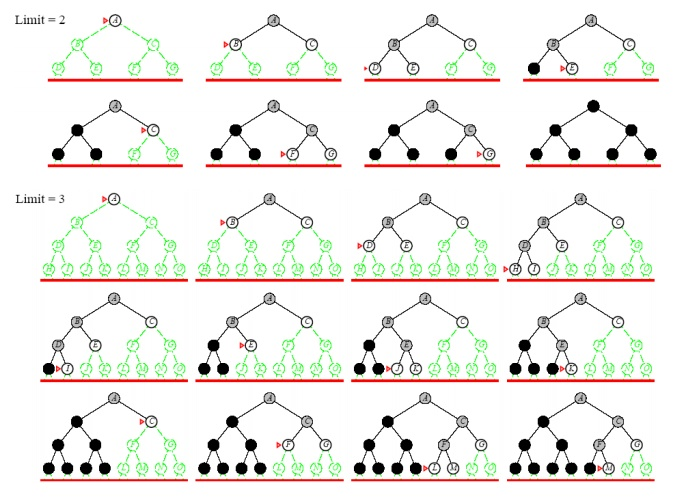Properties of Iterative Deepening

Search:

ŌĆó                      Complete

ŌĆō  Yes

ŌĆó                      Time : N(IDS)=(d)b+(d-1)b2+ŌĆ”ŌĆ”ŌĆ”ŌĆ”+(1)bd

ŌĆō  O(bd)

ŌĆó                      Space

ŌĆō  O(bd)

ŌĆó                      Optimal

ŌĆō  Yes if step cost = 1

ŌĆō  Can be modified to explore uniform cost tree

ŌĆó                      This method is preferred for large state space and when the depth of the search is not known.

ŌĆó                      Memory requirements are modest.

ŌĆó                      Like BFS it is complete

Many states are expanded multiple times.

Lessons from Iterative Deepening Search

ŌĆó                   If branching factor is b and solution is at depth d, then nodes at depth d are generated once, nodes at depth d-1 are generated twice, etc.

ŌĆō  Hence bd + 2b(d-1) + ... + db <= bd / (1 - 1/b)2 = O(bd).

ŌĆō If b=4, then worst case is 1.78 * 4d, i.e., 78% more nodes searched than exist at depth d (in the worst case).

ŌĆó                   Faster than BFS even though IDS generates repeated states

ŌĆō  BFS generates nodes up to level d+1

ŌĆō  IDS only generates nodes up to level d

ŌĆó                   In general, iterative deepening search is the preferred uninformed search method when there is a large search space and the depth of the solution is not known

Example: Route finding problem

Given: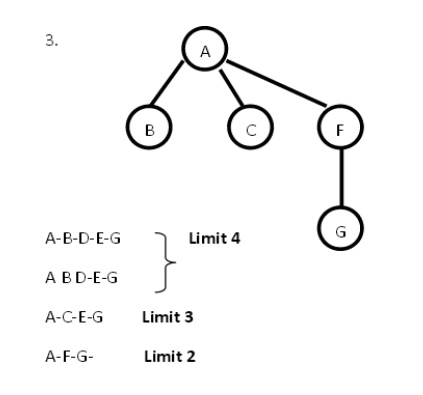Answer: Since it is a IDS tree the lowest depth limit (i.e.) A-F-G is selected as the solution path.

BI-DIRECTIONAL SEARCH

Definition:

It is a strategy that simultaneously searches both the directions (i.e) forward from the initial state and backward from the goal state and stops when the two searches meet in the Middle.ŌĆó                      Alternate searching from the start state toward the goal and from the goal state toward the start.

ŌĆó                      Stop when the frontiers intersect.

ŌĆó                      Works well only when there are unique start and goal states.

ŌĆó                      Requires the ability to generate ŌĆ£predecessorŌĆØ states.

ŌĆó                      Can (sometimes) lead to finding a solution more quickly.

Properties of Bidirectional Search:

1.                          Time Complexity: O(b d/2)

2.                            Space Complexity: O(b d/2)

3.                          Complete: Yes

4.                          Optimal: Yes

Reduce time complexity and space complexity

The space requirement is the most significant weakness of bi-directional search.If two searches do not meet at all, complexity arises in the search technique. In backward search calculating predecessor is difficult task. If more than one goal state exists then explicitly, multiple state searches are required.

COMPARING UNINFORMED SEARCH STRATEGIESŌĆó                   Completeness

ŌĆō  Will a solution always be found if one exists?

ŌĆó                   Time

ŌĆō  How long does it take to find the solution?

ŌĆō Often represented as the number of nodes searched

ŌĆó                      Space

ŌĆō  How much memory is needed to perform the search?

ŌĆō  Often represented as the maximum number of nodes stored at once

ŌĆó                      Optimal

ŌĆō  Will the optimal (least cost) solution be found?

ŌĆó                    Time and space complexity are measured in

ŌĆō  b ŌĆō maximum branching factor of the search tree

ŌĆō  m ŌĆō maximum depth of the state space

ŌĆō  d ŌĆō depth of the least cost solution

Study Material, Lecturing Notes, Assignment, Reference, Wiki description explanation, brief detail
Artificial Intelligence : Uninformed Search Strategies |